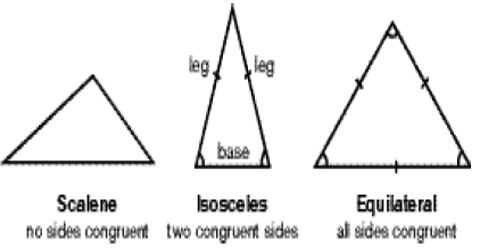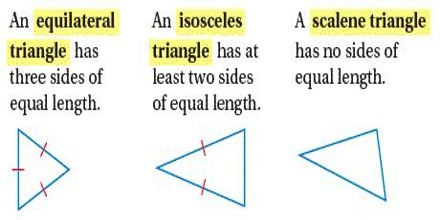Mathematic

# How to Identify Types of Triangles by Sides?Identifying Types of Triangles by Sides

The triangle is perhaps most common shape ever studied in geometry. It is a kind of polygon which has three sides. A triangle eventually has three vertices as well. Types of triangles based on their sides

• An EQUILATERAL triangle has all three sides the same length.
• An ISOSCELES triangle has two sides with the same length.
• A SCALENE triangle has all three sides different lengths.Equilateral triangle: The Equilateral triangle shown on the left has three equal sides and three equal angles. Equilateral triangle-a triangle with exactly 3 congruent sides. Each angle is 60°.In the above triangle, all the sides of triangle denoted by ‘a’ are equal and angles are equal to 60°. So, the above geometrical figure represents a equilateral triangle.

Isosceles Triangle: The Isosceles triangle shown on the left has two equal sides and two equal angles. Isosceles triangle-a triangle with at least 2 congruent sides (i.e. 2 or 3 congruent sides).In the above triangle, sides ‘a’ are equal and angle ‘x’ are also equal. Hence it is an isosceles triangle.

Scalene Triangle: The Scalene Triangle has no congruent sides. In other words, each side must have a different length. Scalene triangle-a triangle with no congruent sides.In the above triangle, all the sides of triangle denoted by a, b and c are unequal and angles x, y and z are also unequal. Hence, the above geometrical figure represents a scalene triangle.